# Van de Graaff Generator - Working Principle

A Van de Graaff generator is an electrostatic generator, invented by an American physicist Robert J. Van de Graaff. It uses a moving belt that accumulates charge on a hollow metal structure designed like a globe, placed on the top of a column that is insulating in nature and thus, creating a very high electric potential in the order of a few million volts.  This results in a very large electric field which is used to accelerate charged particles.

## Working principle of Van de Graaff Generator

Let us consider a large spherical shell of radius R. If we place a charge of magnitude Q on such a sphere, the charge will spread uniformly over the surface of the sphere and the electric field inside the sphere will be equal to zero, and that outside the sphere will be due to the charge Q placed at the centre of the sphere.

So the potential outside is that of a point charge; and inside it is constant, namely the value at the radius R. We thus have:

Potential inside conducting spherical shell of radius R carrying charge Q = constant and is given by,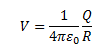Let a small sphere be placed at the center of the large one such that the radius of the smaller sphere is r and the charge over its surface is q. The potential energy thus generated due to the smaller surface at different points in the system can be given as the following values,

At the surface of the small sphere: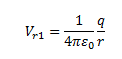At the large spherical shell of radius R: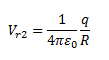If we consider the total charges in the system, that is, q and Q, then the total potential energy due to the system of charges can be given as,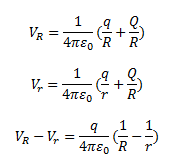Assuming that q is positive, the inner sphere is always at a higher potential and is independent of the charge Q that is accumulated on the larger surface. The difference in potential given by the value V(r)-V(R) is positive. The potential due to Q is constant up to radius R and thus, the difference gets canceled out. If we connect the smaller and the larger sphere with a conducting wire, the charge q, however small, on the smaller sphere gets transferred to the bigger sphere. Here, if we introduce a small charged sphere into a larger spherical shell, as shown in the system, the charge on the larger sphere keeps increasing. Similarly, the potential at the larger sphere would also keep rising as the charge increases, until the breakdown field of air is reached.  The Van de Graaff generator works on the same principle.

Following is the table of link related to the difference between motor and a generator: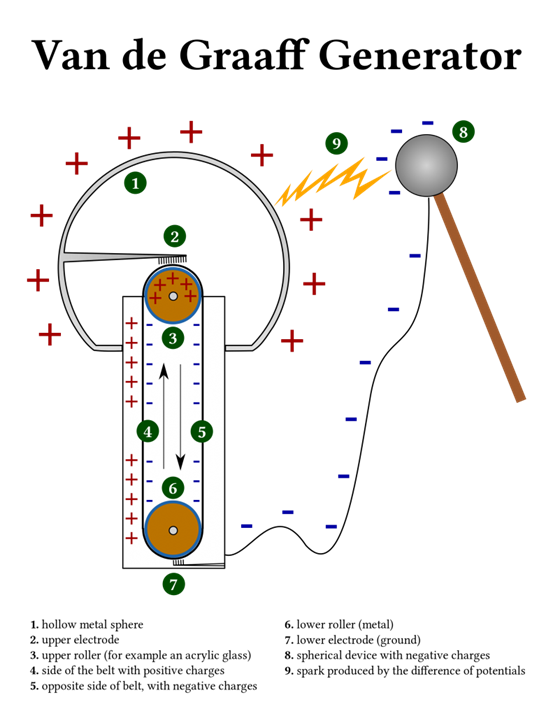In the figure given above, we can see a Van de Graaff generator. Here, a large spherical shell is held at a height of several meters above the ground supported by an insulating column. Two pulleys are wound with a belt-like insulating material, with one being at ground level and the other one at the center of the shell. This belt undertakes a continuous motion, thus carrying a positive charge continuously from the ground to the top.  This belt is kept moving continuously by a motor driving the lower pulley. The positive charge is transferred to the larger shell by a carbon brush, thus rendering the outer shell with a very high potential over the time.